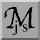jsMath (Test)

If your browser supports JavaScript, be sure it is enabled.

# jsMath Image Mode Test Page

If you see typeset mathematics below, then jsMath Image Mode is working. If you see TeX code instead, jsMath Image Mode is not working for you.

\left(\, \sum_{k=1}^n a_k b_k \right)^2 \le \left(\, \sum_{k=1}^n a_k^2 \right) \left(\, \sum_{k=1}^n b_k^2 \right)

\warning{       jsMath image mode is not working!       }

If the mathematics does not show up properly, you may not have not installed the jsMath image fonts file correctly. Follow the instructions on the linked page.

Once you have jsMath working, go on to the sample page to see if easy/load.js is configured properly.jsMath web pages Created: 14 Feb 2007 Last modified: Jun 16, 2007 8:56:17 AM Comments to: dpvc@union.edu